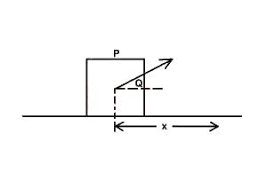# A force F is applied to a body P as shown in the diagram above. If the body P moves through a distance r, which of the following represents the work done?

Question 1

A force F is applied to a body P as shown in the diagram above. If the body P moves through a distance r, which of the following represents the work done?